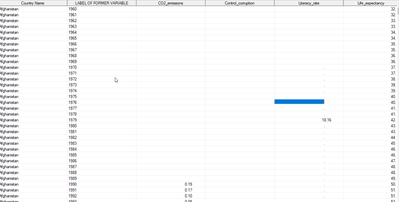## convert \$50. to numeric

Hello I want to convert \$50. to numeric. this is the dataset. when I run proc corr I have a error in the log "in list does not match type prescribed for this list"1 ACCEPTED SOLUTION

Accepted Solutions

## Re: convert \$50. to numeric

The INPUT function is the preferred method, but here are a few more considerations.

First, you will need new variable names.  Existing character variables cannot be turned into numeric variables.  You could conceivably jump through some hoops to re-use the old names, which could look like this:

``````data want;
set have;
numvar = input(charvar, 20.);
drop charvar;
rename numvar = charvar;
run;
``````

That would give you the original variable name as a numeric variable, but it's a tedious process to go through if you have many variables.

Second, you can trade off complexities of the programming if you are willing to tolerate notes in the log:

``````data want;
set have;
length numvar 8;
numvar = charvar;
run;``````

That gives you NUMVAR as the numeric version of CHARVAR, but adds a note to the log about character to numeric conversion.

Finally, if you use the INPUT function, do not add number of decimal places:

``````data want;
set have;
numvar = input(charvar, 6.4);     /* gives the wrong result */
run;``````

Just supply the width to the INPUT function, not the number of positions after the decimal point.

3 REPLIES 3

## Re: convert \$50. to numeric

Use the Input Function with an appropriate informat.

## Re: convert \$50. to numeric

The INPUT function is the preferred method, but here are a few more considerations.

First, you will need new variable names.  Existing character variables cannot be turned into numeric variables.  You could conceivably jump through some hoops to re-use the old names, which could look like this:

``````data want;
set have;
numvar = input(charvar, 20.);
drop charvar;
rename numvar = charvar;
run;
``````

That would give you the original variable name as a numeric variable, but it's a tedious process to go through if you have many variables.

Second, you can trade off complexities of the programming if you are willing to tolerate notes in the log:

``````data want;
set have;
length numvar 8;
numvar = charvar;
run;``````

That gives you NUMVAR as the numeric version of CHARVAR, but adds a note to the log about character to numeric conversion.

Finally, if you use the INPUT function, do not add number of decimal places:

``````data want;
set have;
numvar = input(charvar, 6.4);     /* gives the wrong result */
run;``````

Just supply the width to the INPUT function, not the number of positions after the decimal point.

## Re: convert \$50. to numeric

If the number has 50 digits, converting it to numeric will cause loss of precision.

Discussion stats
• 3 replies
• 445 views
• 2 likes
• 4 in conversation